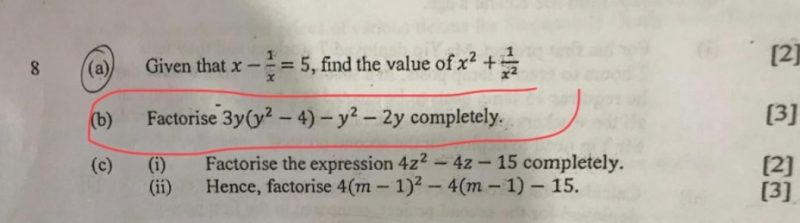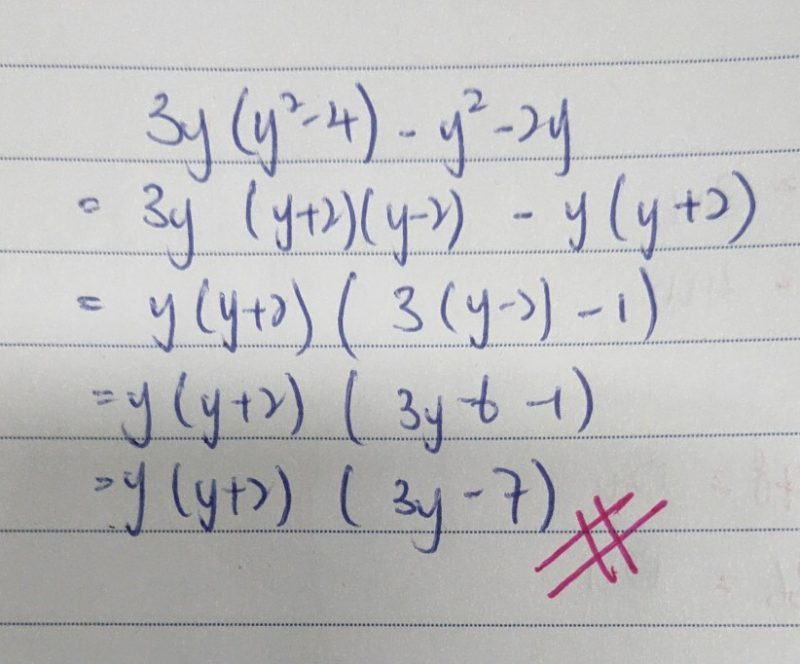# Question**Dear Teachers, pls help to solve 8b. Thank you.

To factorise completely, first expand the expression.

You will get a cubic expression: 3y^{2} – y^{2} – 14y

then take out y

y ( 3y^{2} – y – 14) = y( (3y-7)(y +2) ) (equivalent to factorising a quadratic expression)

“You will get a cubic expression: 3y^{2} – y^{2} – 14y.”

A cubic equation is an equation which can be represented in the form ax^3+bx^2+cx+d=0, where a, b, c, d can be any number (may be complex if given so) where a ≠ 0. An cubic equation has 3 roots (solutions), may be equal or not equal.

The correct term to be used is quadratic equation, which is an equation of the second degree, meaning it contains at least one term that is squared. The standard form is ax² + bx + c = 0 with a, b, and c being constants, or numerical coefficients, and x is an unknown variable.

0 Replies 0 LikesWebsite